## How To Remove Empty Rows in Excel

What is the best way to remove empty rows in Excel? There is a couple of different ways to do it. One way involves using Visual Basic Applications (VBA) and the other way doesn’t. In this article, we will go through both methods on how to remove empty rows.

# Removing Empty Rows

The first method we will go through does not require VBA. It means this method is most likely easier to follow as no coding is required. However if removing empty rows is repetitive task that you need to do time and time again, you might want to consider creating a VBA for it. With VBA, it means the whole process can be simplified into a click of a button.

To remove empty rows:

1. Apply filter to the whole table by first highlighting the whole column and click Filter. Alternatively use hotkey (CTRL & L) to apply Filter:Note: it is important highlight the entire column first. Because the table is currently separated by empty rows, if we do not select the entire column, Excel will think the table ends at the first empty row. Example: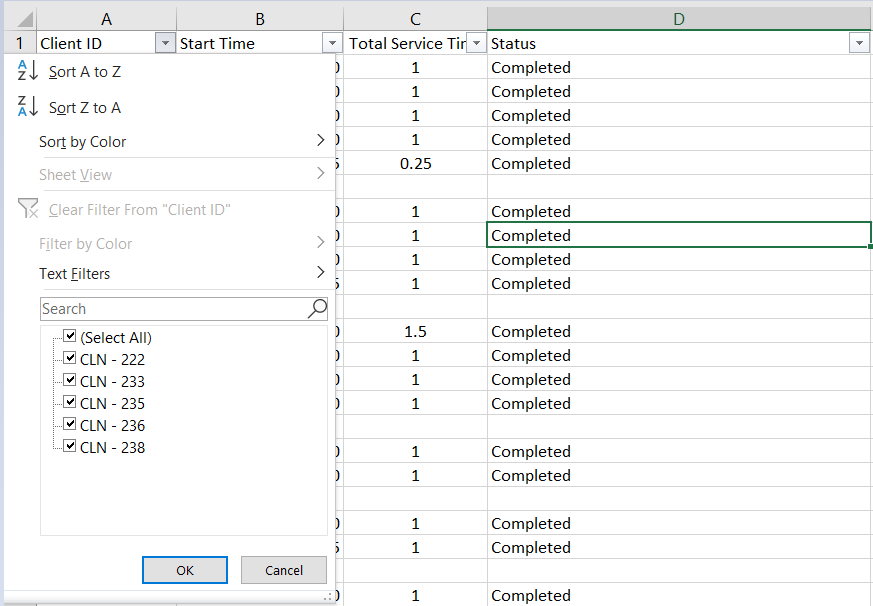2. Filter to (Blanks):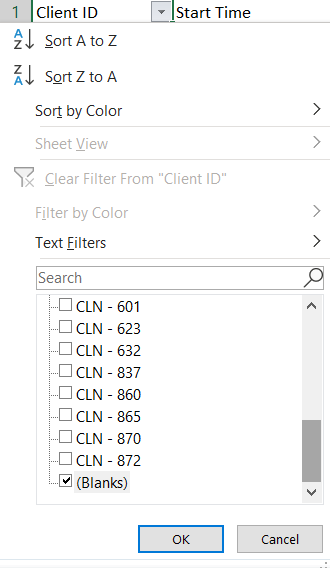3. Highlight all empty rows. You can do this by first:
• Select the first empty row: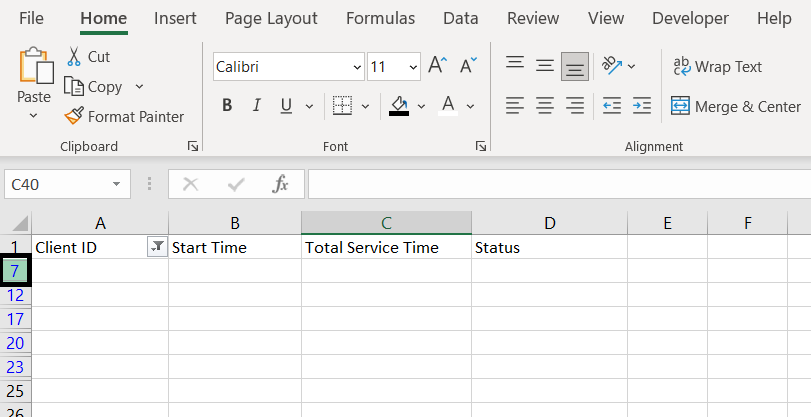• Hold the SHIFT key
• Select the last empty row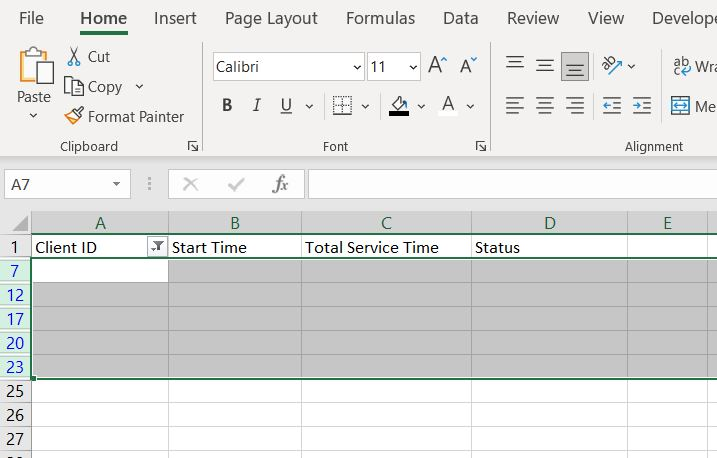4. Right-click and click on Delete Row: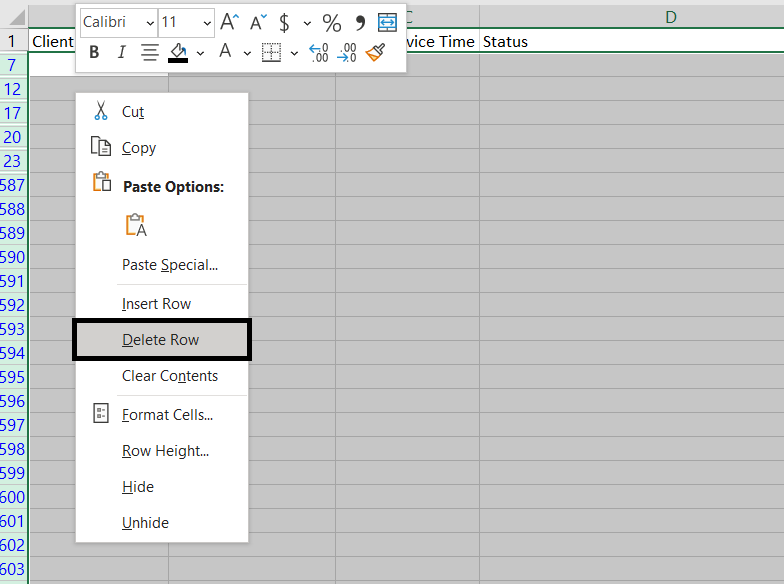5. Remove Filter: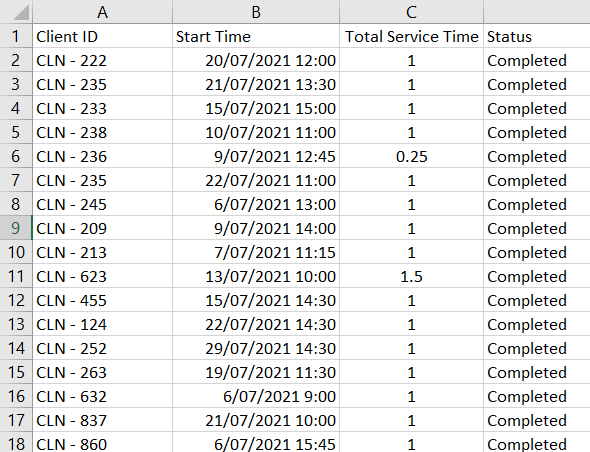Here we can see that all the empty rows in the table are now removed.

# Remove Empty Rows Using VBA

To use VBA, we will first need to make sure Macro/VBA is enabled. Once Macro/VBA is enabled:

1. Go to Developer tab and open Visual Basic
2. Insert a new module
3. Copy and paste following into the module:

A) In this For loop, “For i = 1 to 600” is telling Excel to loop from number 1 to 600 and because we are putting i in Cells(i, 1), we are looping from row 1 to 600. And of course this can change depending on which row our table starts and ends.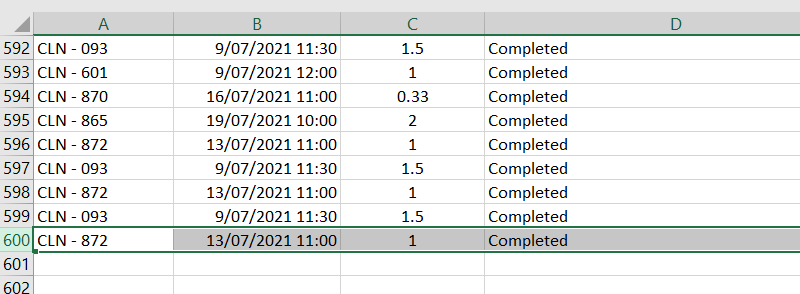In this example the last row of the table is 600 hence we are looping from 1 to 600

B) This number needs to be the total number of items in the column + 1. To find out, highlight the relevant column, check the “Count” at the bottom and + 1.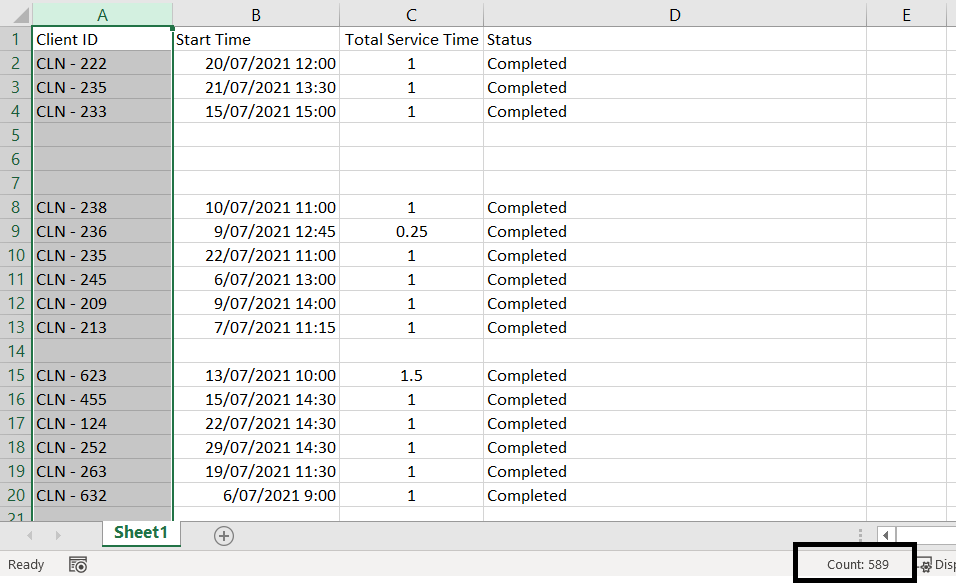In this case, there are 589 items in the column hence we need 590 (589 + 1). Explanation: And this is the row number we need to tell Excel to stop and exit the For loop. This section is here to account for situations where we may have multiple consecutive blank rows. Hence every time a blank row is deleted, instead of moving onto the next row number (next i), we want to check the same row again to see if it is also blank (hence we need i = i – 1). However this can create an endless loop where “i” will never get to 600 if we keep looping i = i – 1. But if we already know that there are only 589 items in the whole column then we can confidently tell Excel to exit the For loop at row 590.